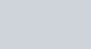Show that the four points
Question:

Show that the four points $A(0,-1,0), B(2,1,-1), C(1,1,1)$ and $D(3,3,0)$ are coplanar. Find the equation of the plane containing them.

Solution: